Question

Petrochemical Production Technology

A) Calculate the minimum pipe thickness based on requirements of ASME B31.3, considering proper Corrosion & Mill Tolerance Allowances, while selecting nominal thickness. The pipe should be designed for crude serve at refinery and having the following data :- ( 3.5 marks )

Design Pressure, P= 800psi

Design Temperature, T=350 F

Outside Diameter, 6.5in

Nominal Pipe Diameter, 6 in

Pipe Schedule ( STD, 5S,10S,XS,XSS ..etc)

Mechanical Allowances inc. Corrosion Allowance,=0..23 in

Allowable Stress, S=15000 psi

Mill Tolerance12.5%

Type of pipe= seamless

B) If the above given data is for a crude line transferred from one of the gathering center at Ahmadi to the Refinery, and needed to be utilized to design the pipe. What would be the minimum wall thickness to allow for a stress of 25,000 psi.( use code B31.4) (2 marks)

C) Analyze the answers for A & B, compare, and state the reasons behind your selection. (Verified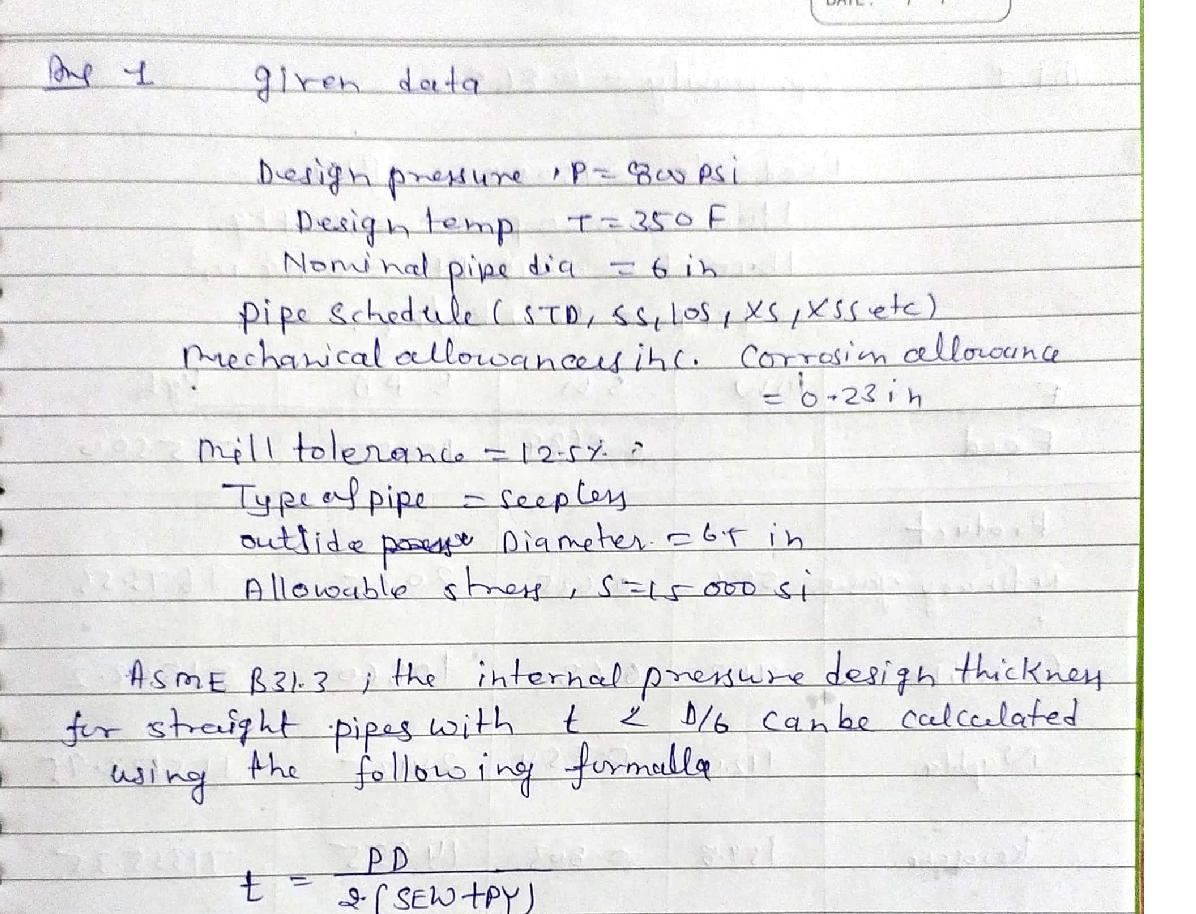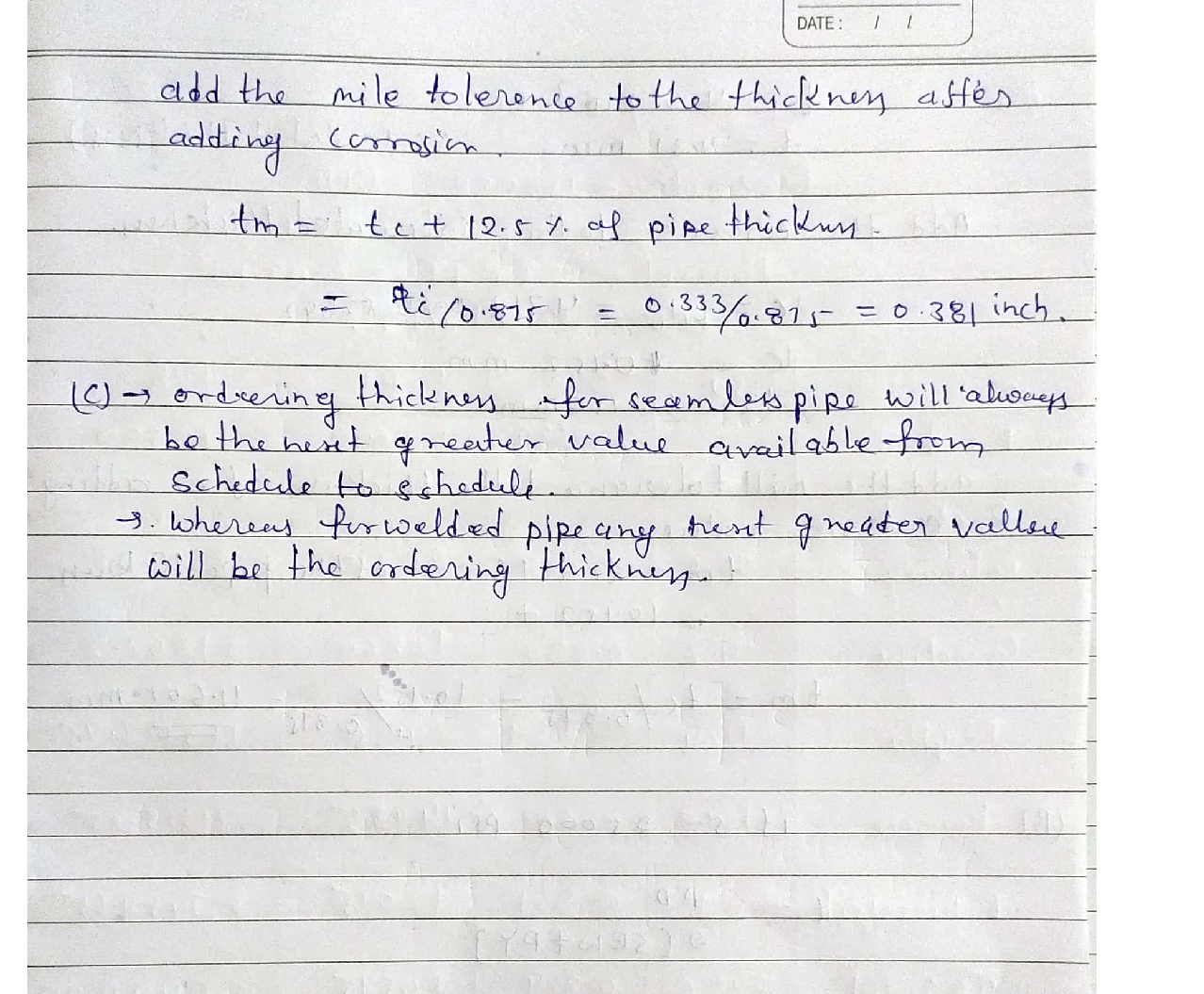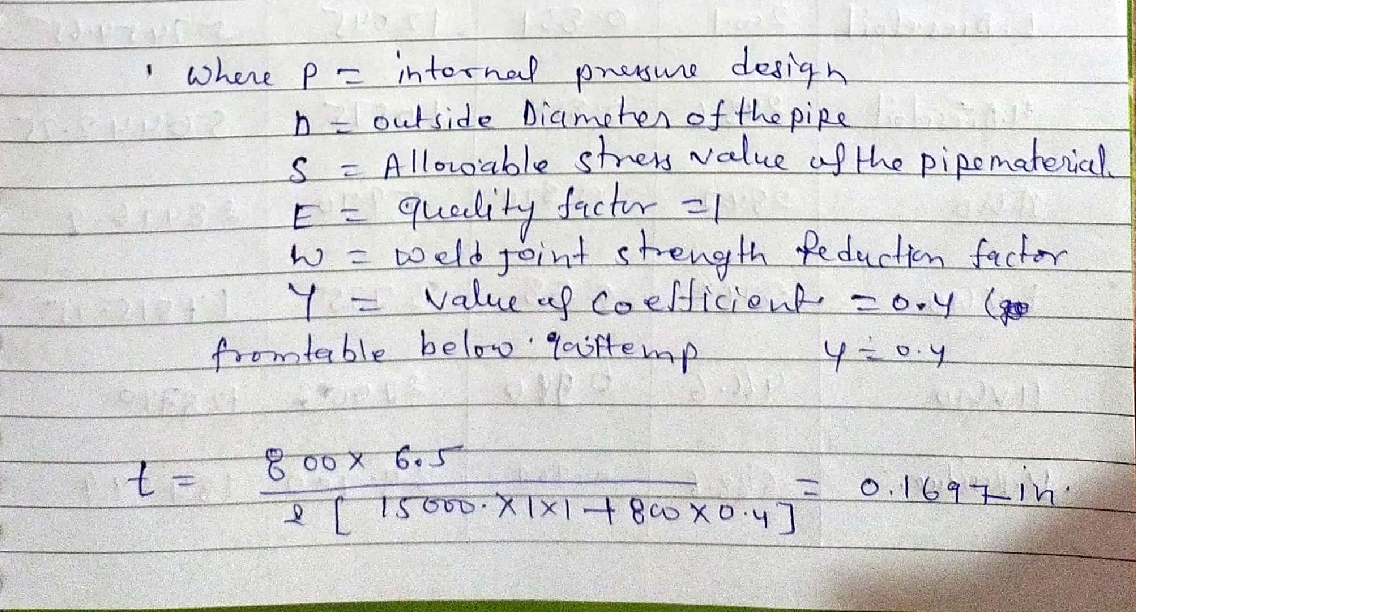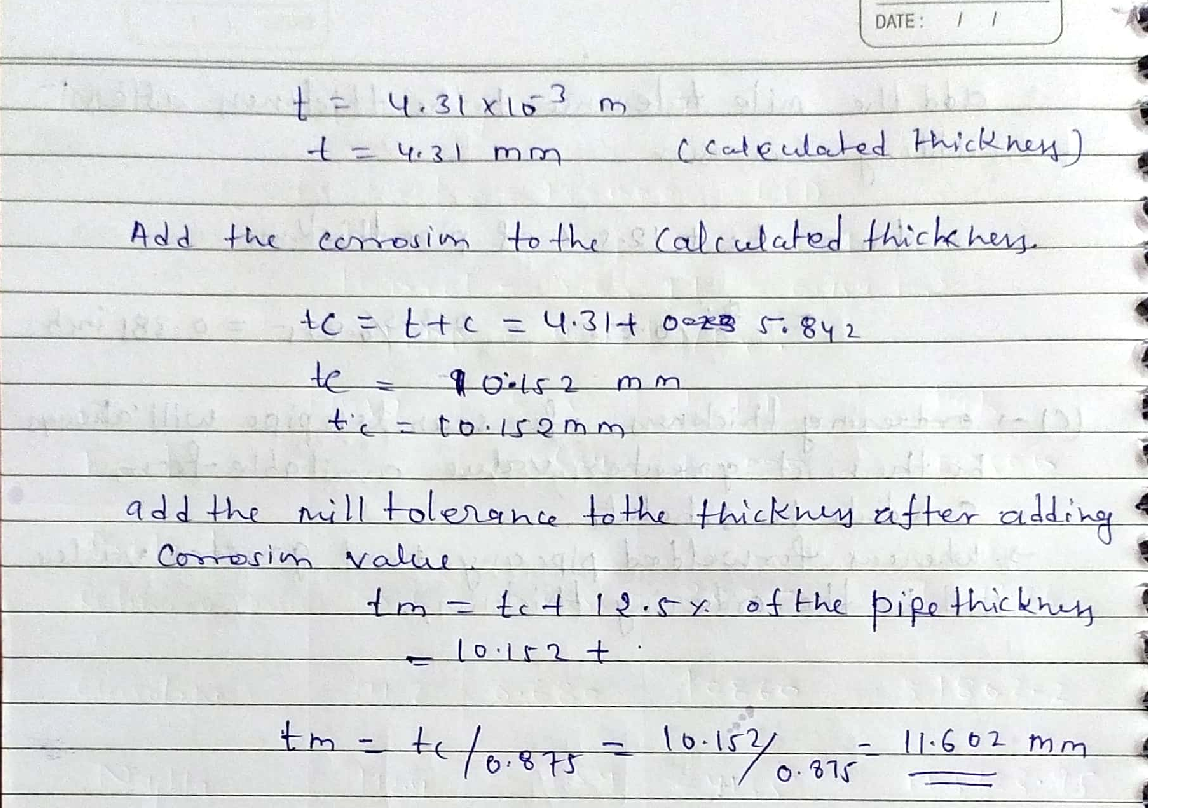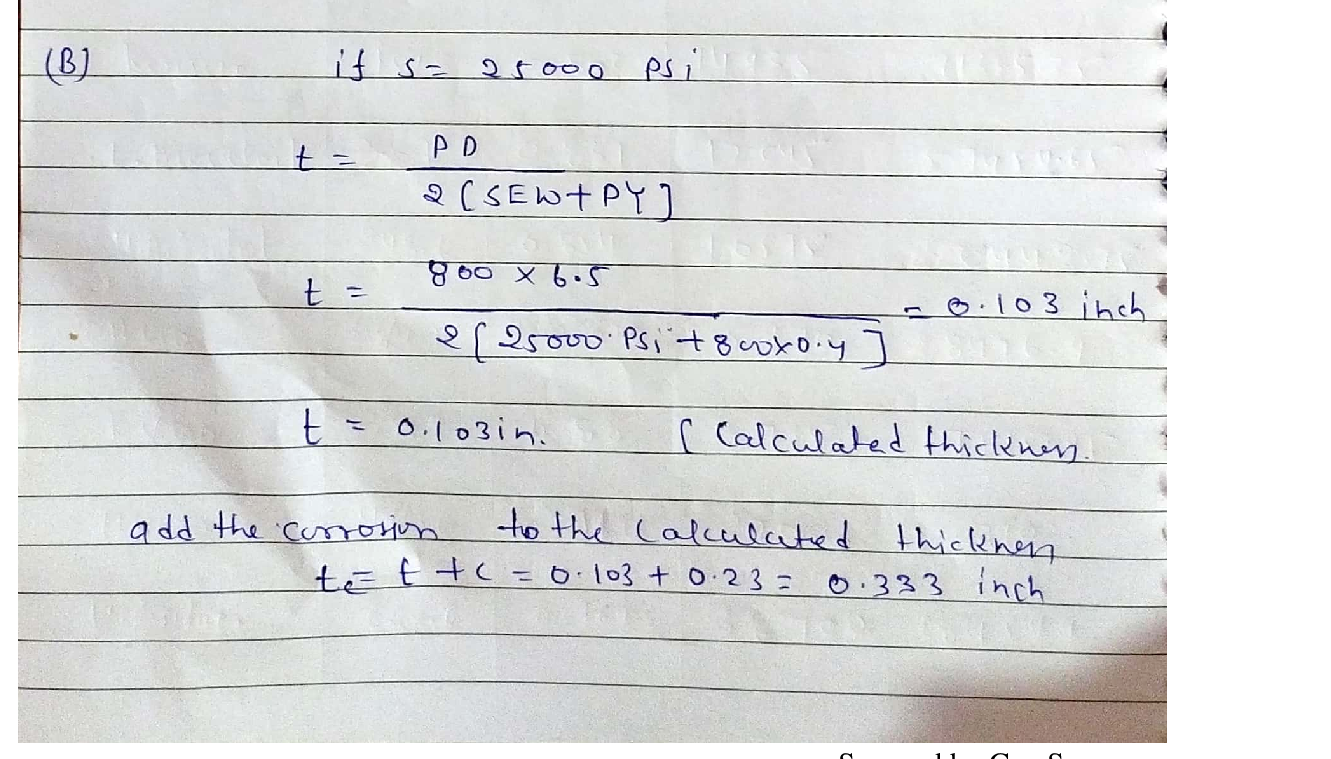### Question 41320Petrochemical Production Technology

The following formation resistivity factors were measured on a suite of gas taken from a producing formation. Determine the coefficient a and the exponent m of this equation. Do appropriate plot. [You can use excel to draw the data, then use your ruler to estimate a & m values, also learn how to list the values by hand on a Log-Log scale]
F=a \phi^{-m}

### Question 41319Petrochemical Production Technology

A consolidated sandstone core sample (d2.1 cm and L = 3.6 cm) is%3|saturated with brine of 0.52 N.m resistivity. The core was desaturated insteps, and the following resistance (N) were measured at each saturation.[You can use excel to draw the data, then use your ruler to estimate the required parameters, also learn how to list the values by hand on a Log-Log scale]
a) Estimate the rock porosityb) Determine the saturation exponent of the rock
b) Determine the saturation exponent, n, of the rock

### Question 41318Petrochemical Production Technology

1.Resistivity of the rock fully saturated with brine is denoted by thesymbol
2.Water resistivity is denoted by the symbol
3.The resistivity in the flushed zone is denoted by the symbol
4.The shoulder bed resistivity is denoted by the symbol
5.The diameter of invasion is denoted by the symbol

### Question 41317Petrochemical Production Technology

1. The saturation exponent.
2. The resistivity of the rock when Sw = 40%.
3. Estimate the resistivity index when the water saturation equals 20%.

### Question 38514Petrochemical Production Technology

A reservoir, with a pattern area of 30 acres, is to be water flooded with a five-spot pattern.The reservoir is 15 ft thick and has a porosity of 0.13 and the initial water saturation is0.38 which is considered immobile. Viscosities of oil and water are 2.3 and 1.1 cp respectively. The residual oil saturation is 0.21. FVF’s for oil and water are assumed to be 1.0. Relative permeability data corresponding to the displacement of oil by water are given in the following equations:
k_{r o}=\left(1-S_{w D}\right)^{242}
k_{n=}=0.78 S_{w D}^{3,8}
where:
S_{w D}=\frac{\left(S_{w}-S_{b w}\right)}{\left(1-S_{o r}-S_{w}\right)}
Assume a pressure drop of 500 psi, K= 0.1 darcy, rw=0.5 ft.
1- Using CGM method and Caudle-Witte approach, predict the performance of the waterflooding.
\text { Plot } N_{p}, W_{i}, W p, q_{t}, q_{o}, q_{w}, f_{w}, \bar{S}_{w}, \text { versus time. }
- At water fractional flow of 0.92, calculate the NPV given the following (OP =40$/STB, WIC=1.08$/STB, WHC = 0.75 \$/STB, DF = 0.09)

### Question 30517Petrochemical Production Technology

Light Naphtha is fed to an isomerisation unit to generate reformate. The volumetric flow rate of light naphtha is 250 m/h with APl equals 70. Determine the following:
a) The yields of each component in the product.t
b) The composition of the product.
\text { Knowing that: } A P I=\frac{141.5}{S G}-131.5

### Question 30516Petrochemical Production Technology

A 200 mol/h of heavy naphtha is introduced to catalytic reformer to produce reformate. The composition of the feed as well as the constants (a,b) for the equilibrium constants K are given for each component in feed in the following table:
Determine the composition of the product at T= 550 °C and pressure 15 bar.

### Question 30515Petrochemical Production Technology

A heavy naphtha is fed to a catalytic reformer at a rate of 271 mol/h. The composition of the heavy naphtha is listed in the following table:
Determine the following:
a) The mole flow for each component in the product of this reformer.
b) The composition of the product from this reformer.

### Question 30514Petrochemical Production Technology

A heavy naphtha feed is introduced to a catalytic reforming unit to generate a reformate. The volumetric flow rate of the heavy naphtha is 200 m'/h. The heavy naphtha comprises 25 vol%naphthene, 70 vol% Paraffins and the remaining is aromatics. The RON of the reformate is 96.Calculate the yields of each product in this reformer.

### Question 30372Petrochemical Production Technology

Consider the following simultaneous nonlinear equations:
x^{2}+y+z=6
x^{2}+y^{2}+z^{2}=14
x y z+z=9
Starting from the initial guesses: xo = 1.5, yo = 2.5 and zo = 3.5, perform the following:
a) Determine the roots of the system of equations using the successive substitution method.Perform three complete iteration. Show your calculations.
b) Determine the roots of the system of equations using the Newton-Raphosn method. Perform the computations until & = 7%. Show your calculations.
c) Write a MATLAB script that performs ten iterations of the Newton-Raphson method.
d) Solve the system of equations by calling the function newtmult, with an accuracy of 10 significant figures.Page 1 of 1

### Submit query

Getting answers to your urgent problems is simple. Submit your query in the given box and get answers Instantly.

### Submit a new QuerySuccess

Assignment is successfully created